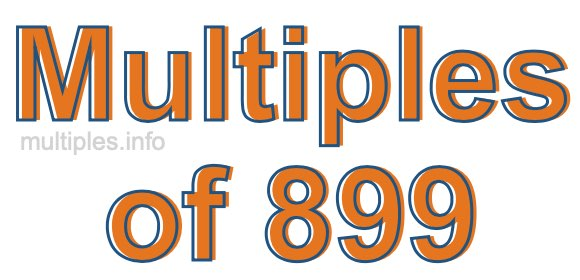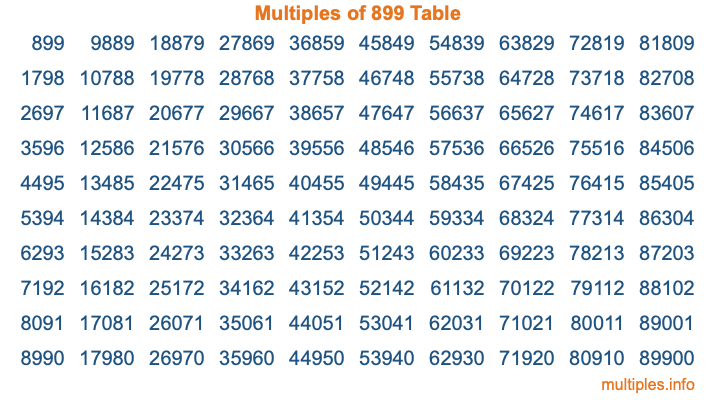Multiples of 899Welcome to the Multiples of 899 page. Here we will first teach you everything you will ever need to know about the multiples of 899, and then give you a study guide summary of everything we taught you to make sure you remember it all. Use this page to look up facts and learn information about the multiples of 899. This page will make you a multiples of eight hundred ninety-nine expert!

Definition of Multiples of 899
Multiples of 899 are all the numbers that when divided by 899 equal an integer. Each of the multiples of 899 are called a multiple. A multiple of 899 is created by multiplying 899 by an integer.

Therefore, to create a list of multiples of 899, you start with 1 multiplied by 899, then 2 multiplied by 899, then 3 multiplied by 899, and so on for as long as you want. Thus, the list of the first five multiples of 899 is 899, 1798, 2697, 3596, and 4495. To see a larger list of multiples of 899, see the printable image of Multiples of 899 further down on this page. We also have a category where you can choose any nth multiple of 899.

Multiples of 899 Checker
The Multiples of 899 Checker below checks to see if any number of your choice is a multiple of 899. In other words, it checks to see if there is any number (integer) that when multiplied by 899 will equal your number. To do that, we divide your number by 899. If the the quotient is an integer, then your number is a multiple of 899.

Is  a multiple of 899?

Least Common Multiple of 899 and ...
A Least Common Multiple (LCM) is the lowest multiple that two or more numbers have in common. This is also called the smallest common multiple or lowest common multiple and is useful to know when you are adding our subtracting fractions. Enter one or more numbers below (899 is already entered) to find the LCM.

Check out our LCM Calculator if you need more details about the Least Common Multiple or if you need the LCM for different numbers for adding and subtraction fractions.

nth Multiple of 899
As we stated above, 899 is the first multiple of 899, 1798 is the second multiple of 899, 2697 is the third multiple of 899, and so on. Enter a number below to find the nth multiple of 899.

th multiple of 899

Multiples of 899 vs Factors of 899
899 is a multiple of 899 and a factor of 899, but that is where the similarities end. All postive multiples of 899 are 899 or greater than 899. All positive factors of 899 are 899 or less than 899.

Below is the beginning list of multiples of 899 and the factors of 899 so you can compare:

Multiples of 899: 899, 1798, 2697, 3596, 4495, etc.

Factors of 899: 1, 29, 31, 899

As you can see, the multiples of 899 are all the numbers that you can divide by 899 to get a whole number. The factors of 899, on the other hand, are all the whole numbers that you can multiply by another whole number to get 899.

It's also interesting to note that if a number (x) is a factor of 899, then 899 will also be a multiple of that number (x).

Multiples of 899 vs Divisors of 899
The divisors of 899 are all the integers that 899 can be divided by evenly. Below is a list of the divisors of 899.

Divisors of 899: 1, 29, 31, 899

The interesting thing to note here is that if you take any multiple of 899 and divide it by a divisor of 899, you will see that the quotient is an integer.

Multiples of 899 Table
Below is an image of the first 100 multiples of 899 in a table. The table is in chronological order, column by column. The first column has the first ten multiples of 899, the second column has the next ten multiples of 899, and so on.The Multiples of 899 Table is also referred to as the 899 Times Table or Times Table of 899. You are welcome to print out our table for your studies.

Negative Multiples of 899
Although not often discussed or needed in math, it is worth mentioning that you can make a list of negative multiples of 899 by multiplying 899 by -1, then by -2, then by -3, and so on, to get the following list of negative multiples of 899:

-899, -1798, -2697, -3596, -4495, etc.

Multiples of 899 Summary
Below is a summary of important Multiples of 899 facts that we have discussed on this page. To retain the knowledge on this page, we recommend that you read through the summary and explain to yourself or a study partner why they hold true.

There are an infinite number of multiples of 899.

A multiple of 899 divided by 899 will equal a whole number.

899 divided by a factor of 899 equals a divisor of 899.

The nth multiple of 899 is n times 899.

The largest factor of 899 is equal to the first positive multiple of 899.

899 is a multiple of every factor of 899.

899 is a multiple of 899.

A multiple of 899 divided by a divisor of 899 equals an integer.

899 divided by a divisor of 899 equals a factor of 899.

Any integer times 899 will equal a multiple of 899.

Multiples of a Number
Here you can get the multiples of another number, all with the same attention to detail as we did for multiples of 899 on this page.

Multiples of
Multiples of 900
Did you find our page about multiples of eight hundred ninety-nine educational? Do you want more knowledge? Check out the multiples of the next number on our list!